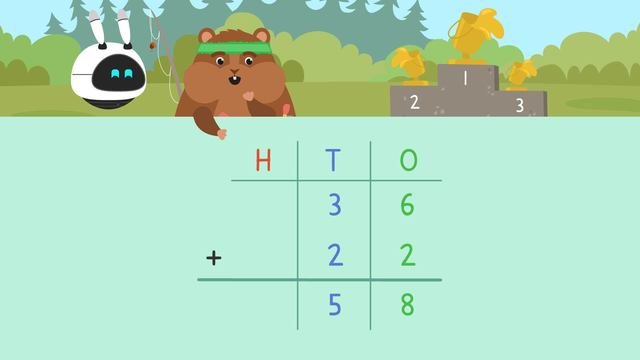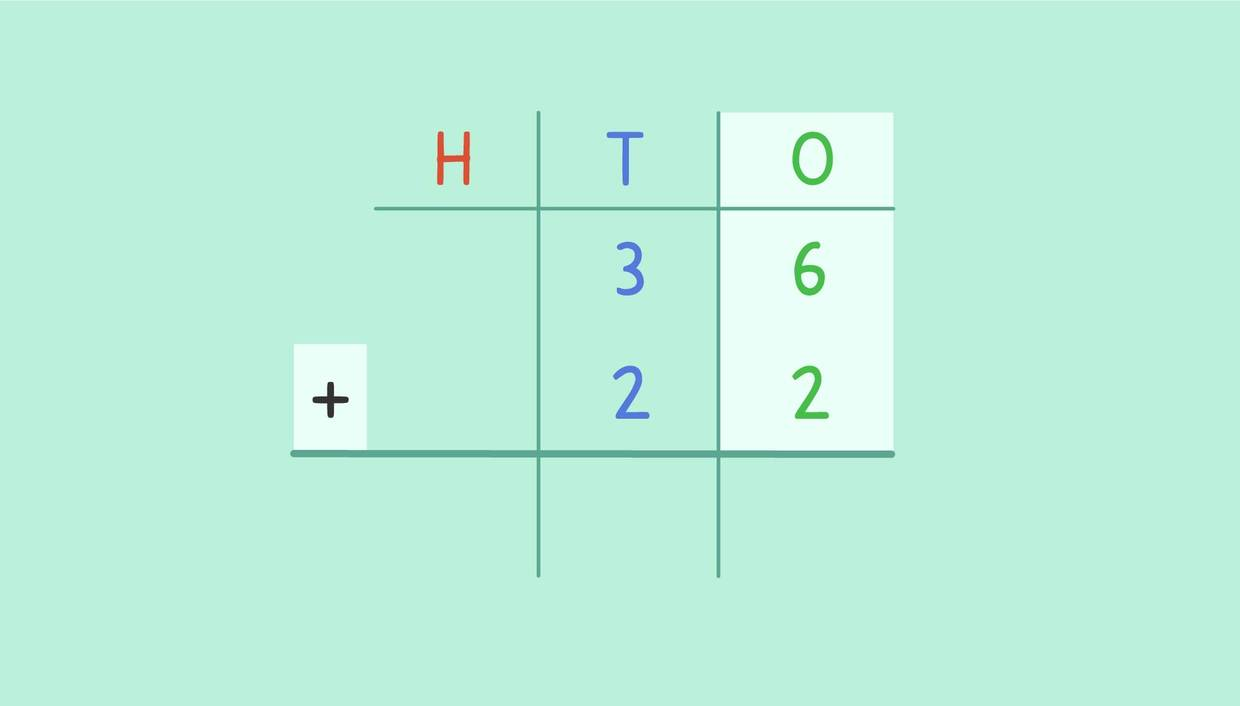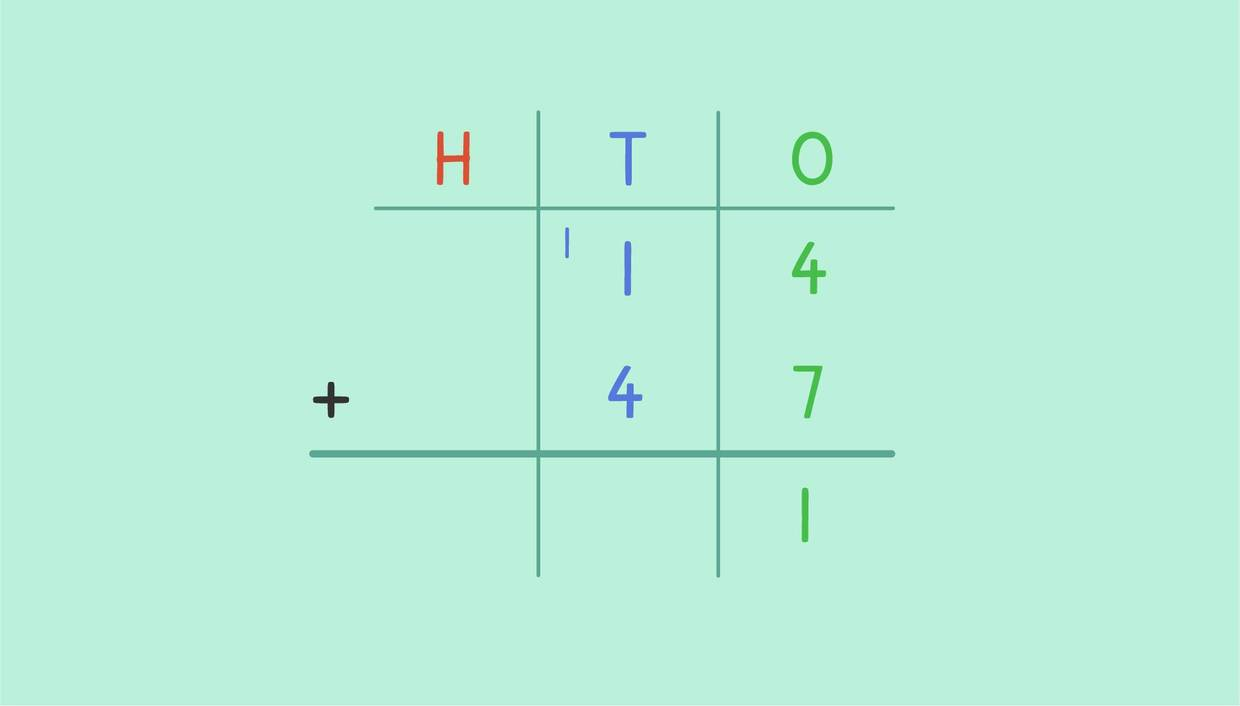# Adding 2 Digit Numbers Using Standard Algorithm

Content Adding 2 Digit Numbers Using Standard AlgorithmRating

Ø 5.0 / 9 ratings
The authorsTeam Digital
Adding 2 Digit Numbers Using Standard Algorithm
CCSS.MATH.CONTENT.4.NBT.B.4

## Basics on the topicAdding 2 Digit Numbers Using Standard Algorithm

Imagine you own 14 toys and your sibling owns 17 toys. Now you want to calculate how many toys you own together! In order to do this, you can use standard algorithm to solve 2 digit addition without regrouping and 2 digit addition with regrouping.

In this text, we will learn how to do 2 digit addition using the standard algorithm.

The standard algorithm is a method to use where the numbers are set up vertically, based on place values. This is an efficient way to find sums when there are several equations you need to solve.

In the explanation, there will be examples that have you adding 2 digit numbers without regrouping as well as adding 2 digit numbers with regrouping.

## Adding Two Digit Numbers – ExampleTo solve this 2-digit addition problem, we set the equation up vertically, with one addend on top of the other in order of their place values. We will find the sum of the numbers by adding the digits from right to left, beginning with the ones place.

What is six plus two more?
Place the eight below the line directly under the ones place.

Next, we move one place over to the left and now we add the tens place.

How many are three tens and two tens? We can write the digit five below the line under the tens place because the five represents five tens or fifty.

The sum is 58.In this problem, we will need to do 2-digit addition with regrouping. We are going to find the sum of fourteen and forty-seven.

Starting with the ones place, four plus seven equals eleven. What do you notice about the sum of the ones place?

Eleven is a two-digit digit number made up of a one and one ten. So, one is written underneath the ones place and one is regrouped on top of the tens place.

Now we add the tens, including this new value added at the top. What is the sum of ten, forty, and ten? Sixty, which means we have six tens.

The sum is 61.

Do you now know how many toys your sibling and you own together? Correct, it is 31 toys in total! For this equation, you needed to regroup a number.

How do you add two digit numbers using the standard algorithm?

Remember: The standard algorithm is a method to use where the numbers are set up vertically, based on place values.

With the standard algorithm you can represent an addition of two numbers neatly to solve it with ease. Have another look at our example addition from earlier:

Tens Ones
1 4
+ 4 7
6 1

At the end of the video, you can find exercises for the continued practice of 2 digit addition as well as a 2 digit addition worksheets. Enjoy!

## Adding 2 Digit Numbers Using Standard Algorithm exercise

Would you like to apply the knowledge you’ve learned? You can review and practice it with the tasks for the video Adding 2 Digit Numbers Using Standard Algorithm.

Hints

Add the digits in the ones column first.

Then add the digits in the tens column.

Solution

Add the digits in the ones column first.

4 + 5 = 9

Then add the digits in the tens column.

3 tens + 2 tens = 5 tens

34 + 29 = 59

• ### Place the digits onto the grid.

Hints

Make sure the ones are in the O column.

The H column represents hundreds.

Solution

We need to make sure the numbers are in the correct column.

If it has 3 digits, it will have a hundred, tens, and ones digit.

310 has 3 hundreds, 1 ten and 0 ones

27 has 2 tens and 7 ones

0 + 7 = 7

1 ten + 2 tens = 3 tens

3 hundreds + 0 hundreds = 3 hundreds

310 + 27 = 337

• ### Calculate the answers to the equations.

Hints

Add up the ones column first.

Make sure if your answer is 2* or more digits, you carry it across.

After adding the ones column, move on to the tens column.

Don't forget to check if there are any numbers that you carried across from the ones column.

Solution

See image for the 1st problem.

For the 2nd problem:

45 + 63 = 108

For the 3rd problem:

44 + 12 = 56

For the 4th problem:

69 + 72 = 141

• ### Match the question to the correct answer.

Hints

Add up all of the digits in the ones column first. If the answer is greater than 9, carry across the extra ten.

If the number in the tens column is greater than 9, make sure you carry across to the hundreds column.

Solution

Using the layout above, work out the answers to the equations.

4. If your answer from the tens column is more than 2 digits, carry across the hundreds digit (the first digit from the number).

38 + 64 = 102 see image above

27 + 35 = 62

39 + 54 = 93

74 + 57 = 131

• ### Identify the place value of the numbers below.

Hints

If the number has two digits and ends in a zero, it is a ten.

If it is a single digit, it is a one.

Solution

In this example, you can see how all the one numbers are in the O column. We have a mixture of words, numerals and Base 10 images.

In the tens column we have a mixture of words, numerals and base 10 images. They all show values of ten

• ### Calculate the answers to the equations.

Hints
Solution

First, add the digits in the ones column.

4 + 7 = 11. 1 ten would go into the tens column with the other tens digits, and the 1 representing the ones would go in the answer box under the ones.

Then, add all of the digits in the tens column.

1 + 8 + 3 = 12

As the answer to this is greater than 9, we need to carry across to the hundreds column.

33 + 42 = 75

18 + 71 = 89

84 + 37 = 121

69 + 33 = 102